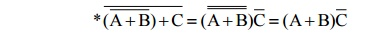Home | | Digital Electronics | Product-of-Sums (POS) Form

# Product-of-Sums (POS) Form

Two or more sum terms multiplied by Boolean multiplication.

PRODUCT-OF-SUMS (POS) FORM:

â€˘               Two or more sum terms multiplied by Boolean multiplication

â€˘               Any expression -> POS using Boolean algebra

â€˘               Examples:

(A+B)(B+C)(A+B+C)

## 1. PRODUCT-OF-SUMS (POS) FORM:

â€˘               Conversion to POS Form:

*              AB+ B(C + D) = B(A + C + D)

*              AB+ ACD = A(B + CD) = A(B + C)(B + D)## 2. IMPLEMENTATION OF POS EXPRESSION:

(A+B)(B+C+D)(A+C)Study Material, Lecturing Notes, Assignment, Reference, Wiki description explanation, brief detail
Digital Electronics : Minimization Techniques and Logic Gates : Product-of-Sums (POS) Form |